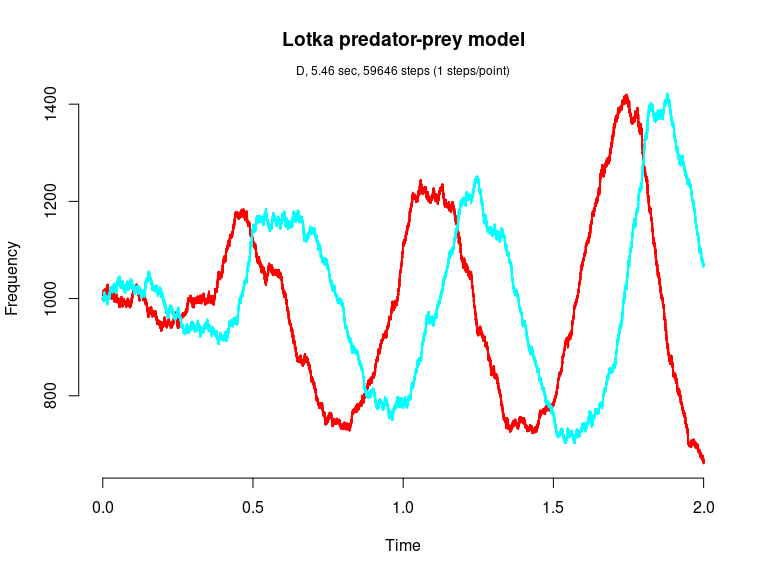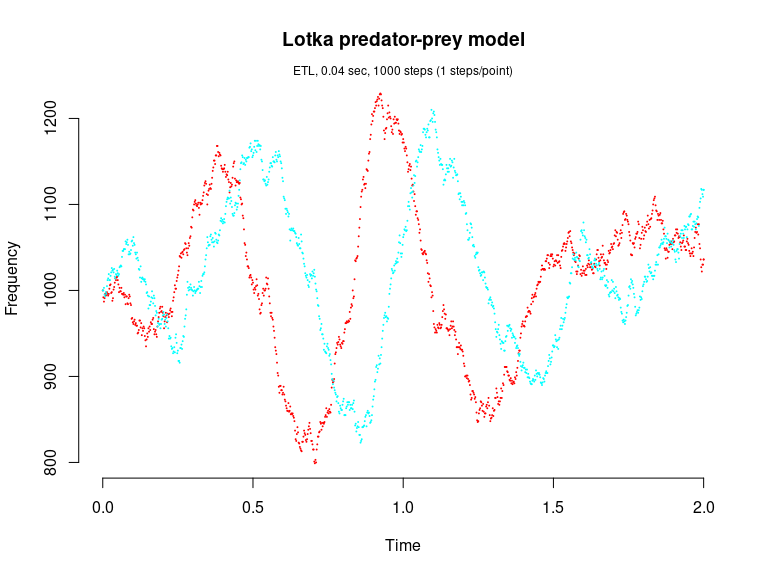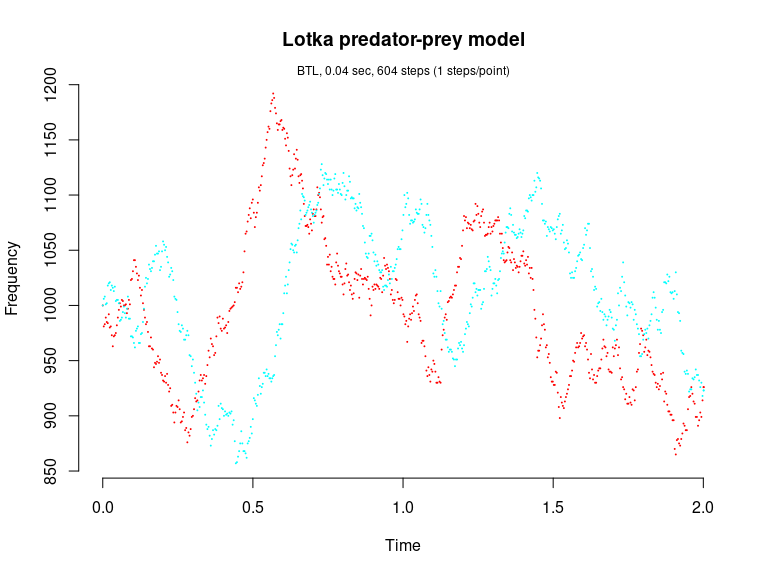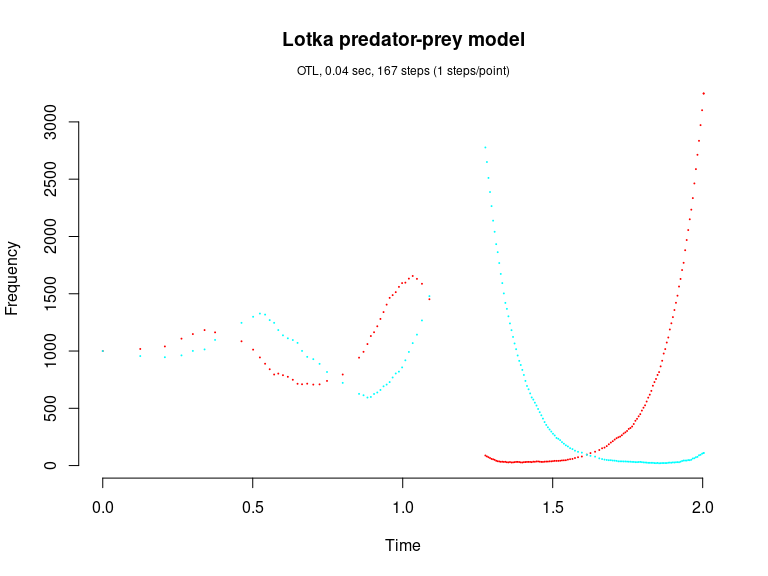# Lotka predator-prey model (Gillespie, 1977; Kot, 2001)

This version of the Lotka predator-prey model is given by

dY1/dt = c1*Y1 - c2*Y1*Y2
dY2/dt = c2*Y1*Y2 - c3*Y2

consisting of the three reaction channels,

      Y1 --c1--> Y1 + Y1
Y1 + Y2 --c2--> Y2 + Y2
Y1 --c3--> 0

library(GillespieSSA)

Define parameters

parms <- c(c1 = 10, c2 = .01, c3 = 10)
tf <- 2                                        # Final time
simName <- "Lotka predator-prey model"         # Name

Define initial state vector

x0 <- c(Y1=1000, Y2=1000)

Define state-change matrix

nu <- matrix(c(+1, -1, 0, 0, 1, -1), nrow = 2, byrow = TRUE)

Define propensity functions

a  <- c("c1*Y1", "c2*Y1*Y2","c3*Y2")

Run simulations with the Direct method

set.seed(1)
out <- ssa(
x0 = x0,
a = a,
nu = nu,
parms = parms,
tf = tf,
method = ssa.d(),
simName = simName,
verbose = FALSE,
consoleInterval = 1
)
ssa.plot(out, show.title = TRUE, show.legend = FALSE)Run simulations with the Explict tau-leap method

set.seed(1)
out <- ssa(
x0 = x0,
a = a,
nu = nu,
parms = parms,
tf = tf,
method = ssa.etl(tau = .002),
simName = simName,
verbose = FALSE,
consoleInterval = 1
)
ssa.plot(out, show.title = TRUE, show.legend = FALSE)Run simulations with the Binomial tau-leap method

set.seed(1)
out <- ssa(
x0 = x0,
a = a,
nu = nu,
parms = parms,
tf = tf,
method = ssa.btl(f = 100),
simName = simName,
verbose = FALSE,
consoleInterval = 1
)
ssa.plot(out, show.title = TRUE, show.legend = FALSE)Run simulations with the Optimized tau-leap method

set.seed(1)
out <- ssa(
x0 = x0,
a = a,
nu = nu,
parms = parms,
tf = tf,
method = ssa.otl(epsilon = .1),
simName = simName,
verbose = FALSE,
consoleInterval = 1
)
ssa.plot(out, show.title = TRUE, show.legend = FALSE)